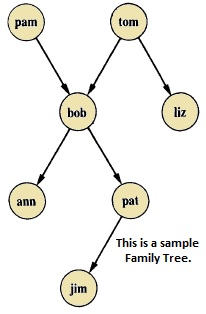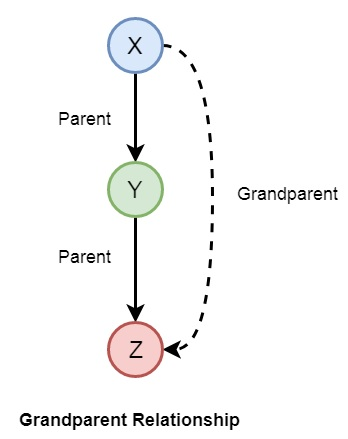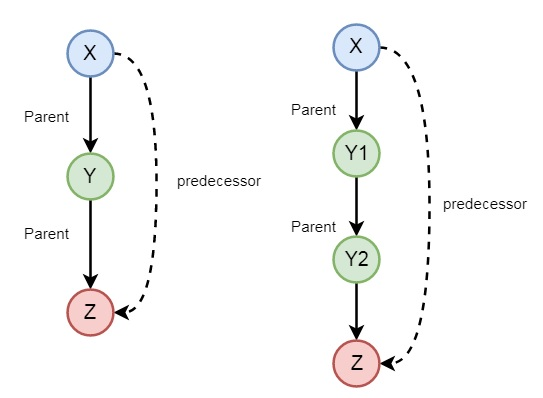Prolog - Relations

Relationship is one of the main features that we have to properly mention in Prolog. These relationships can be expressed as facts and rules. After that we will see about the family relationships, how we can express family based relationships in Prolog, and also see the recursive relationships of the family.

We will create the knowledge base by creating facts and rules, and play query on them.

Relations in Prolog

In Prolog programs, it specifies relationship between objects and properties of the objects.

Suppose, there’s a statement, “Amit has a bike”, then we are actually declaring the ownership relationship between two objects — one is Amit and the other is bike.

If we ask a question, “Does Amit own a bike?”, we are actually trying to find out about one relationship.

There are various kinds of relationships, of which some can be rules as well. A rule can find out about a relationship even if the relationship is not defined explicitly as a fact.

We can define a brother relationship as follows −

Two person are brothers, if,

• They both are male.

• They have the same parent.

Now consider we have the below phrases −

• parent(sudip, piyus).

• parent(sudip, raj).

• male(piyus).

• male(raj).

• brother(X,Y) :- parent(Z,X), parent(Z,Y),male(X), male(Y)

These clauses can give us the answer that piyus and raj are brothers, but we will get three pairs of output here. They are: (piyus, piyus), (piyus, raj), (raj, raj). For these pairs, given conditions are true, but for the pairs (piyus, piyus), (raj, raj), they are not actually brothers, they are the same persons. So we have to create the clauses properly to form a relationship.

The revised relationship can be as follows −

A and B are brothers if −

• A and B, both are male

• They have same father

• They have same mother

• A and B are not same

Family Relationship in Prolog

Here we will see the family relationship. This is an example of complex relationship that can be formed using Prolog. We want to make a family tree, and that will be mapped into facts and rules, then we can run some queries on them.

Suppose the family tree is as follows −Here from this tree, we can understand that there are few relationships. Here bob is a child of pam and tom, and bob also has two children — ann and pat. Bob has one brother liz, whose parent is also tom. So we want to make predicates as follows −

Predicates

• parent(pam, bob).

• parent(tom, bob).

• parent(tom, liz).

• parent(bob, ann).

• parent(bob, pat).

• parent(pat, jim).

• parent(bob, peter).

• parent(peter, jim).

From our example, it has helped to illustrate some important points −

• We have defined parent relation by stating the n-tuples of objects based on the given info in the family tree.

• The user can easily query the Prolog system about relations defined in the program.

• A Prolog program consists of clauses terminated by a full stop.

• The arguments of relations can (among other things) be: concrete objects, or constants (such as pat and jim), or general objects such as X and Y. Objects of the first kind in our program are called atoms. Objects of the second kind are called variables.

• Questions to the system consist of one or more goals.

Some facts can be written in two different ways, like sex of family members can be written in either of the forms −

• female(pam).

• male(tom).

• male(bob).

• female(liz).

• female(pat).

• female(ann).

• male(jim).

Or in the below form −

• sex( pam, feminine).

• sex( tom, masculine).

• sex( bob, masculine).

• … and so on.

Now if we want to make mother and sister relationship, then we can write as given below −In Prolog syntax, we can write −

• mother(X,Y) :- parent(X,Y), female(X).

• sister(X,Y) :- parent(Z,X), parent(Z,Y), female(X), X \== Y.

Now let us see the practical demonstration −

Knowledge Base (family.pl)

female(pam).
female(liz).
female(pat).
female(ann).
male(jim).
male(bob).
male(tom).
male(peter).
parent(pam,bob).
parent(tom,bob).
parent(tom,liz).
parent(bob,ann).
parent(bob,pat).
parent(pat,jim).
parent(bob,peter).
parent(peter,jim).
mother(X,Y):- parent(X,Y),female(X).
father(X,Y):- parent(X,Y),male(X).
haschild(X):- parent(X,_).
sister(X,Y):- parent(Z,X),parent(Z,Y),female(X),X\==Y.
brother(X,Y):-parent(Z,X),parent(Z,Y),male(X),X\==Y.

Output

| ?- [family].
compiling D:/TP Prolog/Sample_Codes/family.pl for byte code...
D:/TP Prolog/Sample_Codes/family.pl compiled, 23 lines read - 3088 bytes written, 9 ms

yes
| ?- parent(X,jim).

X = pat ? ;

X = peter

yes
| ?-
mother(X,Y).

X = pam
Y = bob ? ;

X = pat
Y = jim ? ;

no
| ?- haschild(X).

X = pam ? ;

X = tom ? ;

X = tom ? ;

X = bob ? ;

X = bob ? ;

X = pat ? ;

X = bob ? ;

X = peter

yes
| ?- sister(X,Y).

X = liz
Y = bob ? ;

X = ann
Y = pat ? ;

X = ann
Y = peter ? ;

X = pat
Y = ann ? ;

X = pat
Y = peter ? ;

(16 ms) no
| ?-

Now let us see some more relationships that we can make from the previous relationships of a family. So if we want to make a grandparent relationship, that can be formed as follows −We can also create some other relationships like wife, uncle, etc. We can write the relationships as given below −

• grandparent(X,Y) :- parent(X,Z), parent(Z,Y).

• grandmother(X,Z) :- mother(X,Y), parent(Y,Z).

• grandfather(X,Z) :- father(X,Y), parent(Y,Z).

• wife(X,Y) :- parent(X,Z),parent(Y,Z), female(X),male(Y).

• uncle(X,Z) :- brother(X,Y), parent(Y,Z).

So let us write a prolog program to see this in action. Here we will also see the trace to trace-out the execution.

Knowledge Base (family_ext.pl)

female(pam).
female(liz).
female(pat).
female(ann).

male(jim).
male(bob).
male(tom).
male(peter).

parent(pam,bob).
parent(tom,bob).
parent(tom,liz).
parent(bob,ann).

parent(bob,pat).
parent(pat,jim).
parent(bob,peter).
parent(peter,jim).

mother(X,Y):- parent(X,Y),female(X).
father(X,Y):-parent(X,Y),male(X).
sister(X,Y):-parent(Z,X),parent(Z,Y),female(X),X\==Y.
brother(X,Y):-parent(Z,X),parent(Z,Y),male(X),X\==Y.
grandparent(X,Y):-parent(X,Z),parent(Z,Y).
grandmother(X,Z):-mother(X,Y),parent(Y,Z).
grandfather(X,Z):-father(X,Y),parent(Y,Z).
wife(X,Y):-parent(X,Z),parent(Y,Z),female(X),male(Y).
uncle(X,Z):-brother(X,Y),parent(Y,Z).

Output

| ?- [family_ext].
compiling D:/TP Prolog/Sample_Codes/family_ext.pl for byte code...
D:/TP Prolog/Sample_Codes/family_ext.pl compiled, 27 lines read - 4646 bytes written, 10 ms

| ?- uncle(X,Y).

X = peter
Y = jim ? ;

no
| ?- grandparent(X,Y).

X = pam
Y = ann ? ;

X = pam
Y = pat ? ;

X = pam
Y = peter ? ;

X = tom
Y = ann ? ;

X = tom
Y = pat ? ;

X = tom
Y = peter ? ;

X = bob
Y = jim ? ;

X = bob
Y = jim ? ;

no
| ?- wife(X,Y).

X = pam
Y = tom ? ;

X = pat
Y = peter ? ;

(15 ms) no
| ?-

Tracing the output

In Prolog we can trace the execution. To trace the output, you have to enter into the trace mode by typing “trace.”. Then from the output we can see that we are just tracing “pam is mother of whom?”. See the tracing output by taking X = pam, and Y as variable, there Y will be bob as answer. To come out from the tracing mode press “notrace.”

Program

| ?- [family_ext].
compiling D:/TP Prolog/Sample_Codes/family_ext.pl for byte code...
D:/TP Prolog/Sample_Codes/family_ext.pl compiled, 27 lines read - 4646 bytes written, 10 ms

(16 ms) yes
| ?- mother(X,Y).

X = pam
Y = bob ? ;

X = pat
Y = jim ? ;

no
| ?- trace.
The debugger will first creep -- showing everything (trace)

yes
{trace}
| ?- mother(pam,Y).
1 1 Call: mother(pam,_23) ?
2 2 Call: parent(pam,_23) ?
2 2 Exit: parent(pam,bob) ?
3 2 Call: female(pam) ?
3 2 Exit: female(pam) ?
1 1 Exit: mother(pam,bob) ?

Y = bob

(16 ms) yes
{trace}
| ?- notrace.
The debugger is switched off

yes
| ?-

Recursion in Family Relationship

In the previous section, we have seen that we can define some family relationships. These relationships are static in nature. We can also create some recursive relationships which can be expressed from the following illustration −So we can understand that predecessor relationship is recursive. We can express this relationship using the following syntax −

predecessor(X, Z) :- parent(X, Z).
predecessor(X, Z) :- parent(X, Y),predecessor(Y, Z).

Now let us see the practical demonstration.

Knowledge Base (family_rec.pl)

female(pam).
female(liz).
female(pat).
female(ann).

male(jim).
male(bob).
male(tom).
male(peter).

parent(pam,bob).
parent(tom,bob).
parent(tom,liz).
parent(bob,ann).
parent(bob,pat).
parent(pat,jim).
parent(bob,peter).
parent(peter,jim).

predecessor(X, Z) :- parent(X, Z).
predecessor(X, Z) :- parent(X, Y),predecessor(Y, Z).

Output

| ?- [family_rec].
compiling D:/TP Prolog/Sample_Codes/family_rec.pl for byte code...
D:/TP Prolog/Sample_Codes/family_rec.pl compiled, 21 lines read - 1851 bytes written, 14 ms

yes
| ?- predecessor(peter,X).

X = jim ? ;

no
| ?- trace.
The debugger will first creep -- showing everything (trace)

yes
{trace}
| ?- predecessor(bob,X).
1 1 Call: predecessor(bob,_23) ?
2 2 Call: parent(bob,_23) ?
2 2 Exit: parent(bob,ann) ?
1 1 Exit: predecessor(bob,ann) ?

X = ann ? ;
1 1 Redo: predecessor(bob,ann) ?
2 2 Redo: parent(bob,ann) ?
2 2 Exit: parent(bob,pat) ?
1 1 Exit: predecessor(bob,pat) ?

X = pat ? ;
1 1 Redo: predecessor(bob,pat) ?
2 2 Redo: parent(bob,pat) ?
2 2 Exit: parent(bob,peter) ?
1 1 Exit: predecessor(bob,peter) ?

X = peter ? ;
1 1 Redo: predecessor(bob,peter) ?
2 2 Call: parent(bob,_92) ?
2 2 Exit: parent(bob,ann) ?
3 2 Call: predecessor(ann,_23) ?
4 3 Call: parent(ann,_23) ?
4 3 Fail: parent(ann,_23) ?
4 3 Call: parent(ann,_141) ?
4 3 Fail: parent(ann,_129) ?
3 2 Fail: predecessor(ann,_23) ?
2 2 Redo: parent(bob,ann) ?
2 2 Exit: parent(bob,pat) ?
3 2 Call: predecessor(pat,_23) ?
4 3 Call: parent(pat,_23) ?
4 3 Exit: parent(pat,jim) ?
3 2 Exit: predecessor(pat,jim) ?
1 1 Exit: predecessor(bob,jim) ?

X = jim ? ;
1 1 Redo: predecessor(bob,jim) ?
3 2 Redo: predecessor(pat,jim) ?
4 3 Call: parent(pat,_141) ?
4 3 Exit: parent(pat,jim) ?
5 3 Call: predecessor(jim,_23) ?
6 4 Call: parent(jim,_23) ?
6 4 Fail: parent(jim,_23) ?
6 4 Call: parent(jim,_190) ?
6 4 Fail: parent(jim,_178) ?
5 3 Fail: predecessor(jim,_23) ?
3 2 Fail: predecessor(pat,_23) ?
2 2 Redo: parent(bob,pat) ?
2 2 Exit: parent(bob,peter) ?
3 2 Call: predecessor(peter,_23) ?
4 3 Call: parent(peter,_23) ?
4 3 Exit: parent(peter,jim) ?
3 2 Exit: predecessor(peter,jim) ?
1 1 Exit: predecessor(bob,jim) ?

X = jim ?

(78 ms) yes
{trace}
| ?-

Useful Video Courses

Video

Prolog Online Training

65 Lectures 5 hours

Video